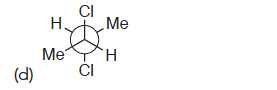# Problem: Each of the following compounds possesses a plane of symmetry. Find the plane of symmetry in each compound. In some cases, you will need to rotate a single bond to place the molecule into a conformation where you can more readily see the plane of symmetry.

###### FREE Expert Solution
100% (173 ratings)
###### Problem Details

Each of the following compounds possesses a plane of symmetry. Find the plane of symmetry in each compound. In some cases, you will need to rotate a single bond to place the molecule into a conformation where you can more readily see the plane of symmetry.What scientific concept do you need to know in order to solve this problem?

Our tutors have indicated that to solve this problem you will need to apply the Test 1: Plane of Symmetry concept. You can view video lessons to learn Test 1: Plane of Symmetry . Or if you need more Test 1: Plane of Symmetry practice, you can also practice Test 1: Plane of Symmetry practice problems.

What is the difficulty of this problem?

Our tutors rated the difficulty ofEach of the following compounds possesses a plane of symmetr...as low difficulty.

How long does this problem take to solve?

Our expert Organic tutor, Jonathan took 2 minutes and 32 seconds to solve this problem. You can follow their steps in the video explanation above.

What professor is this problem relevant for?

Based on our data, we think this problem is relevant for Professor Jones' class at BAYLOR.

What textbook is this problem found in?

Our data indicates that this problem or a close variation was asked in Organic Chemistry - Klein 1st Edition. You can also practice Organic Chemistry - Klein 1st Edition practice problems.